## Monday, August 30, 2010

### F#, Fuzzy Logic, WPF, and Tomatoes!

This is my 100th blog post, and to celebrate, I’m pulling out all the stops. This example will combine F#, fuzzy logic, WPF, and tomatoes!

The example below illustrates a simple fuzzy logic control simulator. In this case, what’s being controlled is the behavior of graphical tomatoes which “chase” the mouse cursor. The fuzzy inference system has two inputs: 1) the distance from the tomato to the cursor, and 2) the speed of the cursor. There is a single output: the speed at which a tomato should chase the cursor. To make things even more interesting, I’ve simulated tomatoes of three dispositions: timid, cautious, and aggressive. The green tomato, being green, is the timid one. The yellow tomato, like the traffic light of the same color, is cautious. The red tomato, like its active color, is aggressive.

Below is the application with the window reduced a bit to fit the blog. In real life, it’s more fun to run it full screen.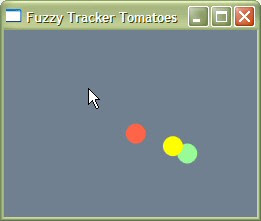This is a WPF application, so it will require a number of additional steps when making it a Visual Studio project:

1) First, create a new F# project of type console and copy and paste the code below. (To make things more convenient, I made the entire project, fuzzy logic included, into one big file. If there are problems with carriage returns and line feeds, try pasting via an intermediate editor such as WordPad.)

2) Second, under project properties, set the application type to “Windows Application.”

3) Third, add the appropriate references. The references for VS2010 and .NET 4.0 are listed in the source code below. For other versions of Visual Studio and .NET, you can use the time honored trial and error technique of letting the compiler complain about the missing references and then adding them.

And that’s it! As always, all the code here is presented "as-is" and without warranty or implied fitness of any kind; use at your own risk.

`// I have the following libraries referenced`
`// in the solution as 4.0 Client Profile.`
`// These may vary for other versions of .NET.`
`//`
`// Accessibility`
`// FSharp.Core`
`// mscorlib`
`// PresentationCore`
`// PresentationFramework`
`// System`
`// System.Core`
`// System.Numerics`
`// System.Xaml`
`// UIAutomationTypes`
`// WindowsBase`
` `
`open System`
`open System.Windows`
`open System.Windows.Controls`
`open System.Windows.Media`
` `
`// Here is the fuzzy logic subsystem.`
` `
`// The input functions are trapezoids.`
`// The precomputations and closures`
`// make them look more complicated than`
`// they really are.`
` `
`// Technically, since Min and Max are`
`// trapezoids with one side at infinity,`
`// a single function would suffice.`
`// But three functions are more efficient`
`// and comprehensible.`
` `
`// Infinite to the left.`
`let inMin x0 x1 =`
`  let m = 1.0/(x0-x1)`
`  let b = 1.0-x0*m `
`  (fun x ->  `
`     match x<=x0 with`
`     | true -> 1.0`
`     | _ -> `
`     match x<=x1 with`
`     | true -> x*m+b`
`     | _ -> 0.0 )`
` `
`// In the middle.`
`let inMid x0 x1 x2 x3 =`
`  let ml = 1.0/(x1-x0)`
`  let mh = 1.0/(x2-x3)`
`  let bl = 1.0-x1*ml`
`  let bh = 1.0-x2*mh`
`  (fun x -> `
`     match x<x0 with`
`     | true -> 0.0`
`     | _ ->`
`     match x<x1 with`
`     | true -> x*ml+bl`
`     | _ ->`
`     match x<=x2 with`
`     | true -> 1.0`
`     | _ ->`
`     match x<=x3 with`
`     | true -> x*mh+bh`
`     | _ -> 0.0 )`
` `
`// Infinite to the right.`
`let inMax x0 x1 =`
`  let m = 1.0/(x1-x0)`
`  let b = 1.0-x1*m `
`  (fun x ->  `
`     match x>=x1 with`
`     | true -> 1.0`
`     | _ -> `
`     match x>=x0 with`
`     | true -> x*m+b`
`     | _ -> 0.0 )`
` `
`// The output set is a symmetric triangle.`
`// This function returns the area and centroid.`
`let outSym xc dx h = `
`  dx*(2.0-h)*h,xc   `
` `
`// Fire one rule.`
`let fire x (inSet,outSet) =`
`  x |> inSet |> outSet`
` `
`// Fire a rule vector.`
`let fireV xl (inSets,outSet) =`
`  Seq.map2 (fun f x->f x) inSets xl `
`  |> Seq.min`
`  |> outSet `
` `
`// Fire and defuzzify a ruleset.`
`let private fireAll0 f sets x =`
`  List.map (f x) sets `
`  |> List.fold (fun (aa,cc)(a,c)->(aa+a,cc+a*c)) (0.0,0.0)`
`  |> (fun (aa,cc)->cc/aa)`
` `
`// Scalar fire and defuzzify a ruleset.`
`let fireAll sets x = `
`  fireAll0 fire sets x`
` `
`// Vector fire and defuzzify a ruleset.`
`let fireAllV sets x = `
`  fireAll0 fireV sets x`
` `
`// Helper functions for this ruleset.`
` `
`let estimate rules distance speed =`
`  (fireAllV rules [distance;speed])`
` `
`let estimateXY rules dX sX dY sY =`
`  estimate rules dX sX,`
`  estimate rules dY sY`
` `
`// Here are the rules.`
`// I got them about 90% of the way`
`// on the first shot just by thinking `
`// about them, and about 10% by`
`// experimentation and tweaking.`
`// That's the power of fuzzy logic!`
` `
`// Distance values in WPF units.`
`let adjacent = inMin 0.0 4.0`
`let near     = inMid 2.0 4.0 10.0 80.0`
`let far      = inMid 10.0 80.0 140.0 200.0`
`let wayOff   = inMax 140.0 200.0`
` `
`// Cursor movement values in WPF units.`
`let still  = inMin 0.0 2.0`
`let medium = inMid 0.0 2.0 10.0 40.0`
`let fast   = inMax 10.0 40.0`
` `
`// Tomato movement values in WPF units.`
`let hold  = outSym 0.0 1.0`
`let creep = outSym 1.0 1.0`
`let walk  = outSym 2.5 1.0`
`let run   = outSym 7.0 1.0`
` `
`// Constant.`
`let setConst h = (fun _->h)`
` `
`// Three basic behaviour types`
`// will be illustrated using `
`// three "tomatoes" of`
`// varying disposition.`
` `
`let rulesTimid = `
`  [`
`    ([adjacent;still], hold);`
`    ([near;    still], creep);`
`    ([far;     still], walk);`
`    ([wayOff;  still], run);`
` `
`    ([(setConst 1.0);medium],creep);`
`    ([(setConst 1.0);fast],hold);`
`  ]`
` `
`let rulesCautious =`
`  [`
`    ([adjacent;still], hold);`
`    ([near;    still], walk);`
`    ([far;     still], walk);`
`    ([wayOff;  still], run);`
` `
`    ([adjacent;medium],hold);`
`    ([near;    medium],walk);`
`    ([far;     medium],walk);`
`    ([wayOff;  medium],run);`
` `
`    ([(setConst 1.0);fast],hold);`
`  ]`
` `
`let rulesAggressive =`
`  [`
`    ([adjacent;still], hold);`
`    ([near;    still], walk);`
`    ([far;     still], run);`
`    ([wayOff;  still], run);`
` `
`    ([adjacent;medium],hold);`
`    ([near;    medium],walk);`
`    ([far;     medium],walk);`
`    ([wayOff;  medium],run);`
` `
`    ([adjacent;fast],walk);`
`    ([near;    fast],walk);`
`    ([far;     fast],run);`
`    ([wayOff;  fast],run);`
`  ]`
` `
` `
`// Tomato record.`
`type Tomato<'Rules> = `
`  {`
`    Shape : Shapes.Ellipse; `
`    Rules : 'Rules;`
`    // These store momentum.`
`    mutable Mx : float; `
`    mutable My : float; `
`  }`
` `
`let makeTomato rules brush =`
`  let shape = new Shapes.Ellipse();`
`  shape.Fill <- brush`
`  { `
`    Shape = shape; `
`    Rules = rules;`
`    Mx = 0.0;`
`    My = 0.0;`
`  }`
` `
`// Define the tomatoes.`
`// This could also be done in MainWindow;`
`// I do it here for ease of reading.`
` `
`let tomatoes = `
`  [`
`    makeTomato rulesTimid Brushes.PaleGreen;`
`    makeTomato rulesCautious Brushes.Yellow;`
`    makeTomato rulesAggressive Brushes.Tomato;`
`  ]`
` `
` `
`// Here is the user interface subsystem.`
` `
`/// WPF main window.`
`type MainWindow (app: Application) =`
`  inherit Window()`
` `
`  let canvas = System.Windows.Controls.Canvas() `
` `
`  /// Momentum factor.`
`  /// Lower = more dampening.`
`  /// This can be fun to play with.`
`  [<Literal>]`
`  let momentum = 0.7`
` `
`  [<Literal>]`
`  let tomatoRadiusX = 10.0`
`  [<Literal>]`
`  let tomatoRadiusY = 10.0`
` `
`  let mutable tomatoTargetLeft = 0.0`
`  let mutable tomatoTargetTop = 0.0`
`  let mutable tomatoTargetLeft0 = 0.0`
`  let mutable tomatoTargetTop0 = 0.0`
` `
`  // This timer handles tomato movement.`
`  let timer = `
`    new System.Windows.Threading.DispatcherTimer()`
` `
`  /// Tomato movement timer callback.`
`  let moveTomato e =`
`    // Target speed.`
`    let sX = tomatoTargetLeft-tomatoTargetLeft0`
`    let sY = tomatoTargetTop-tomatoTargetTop0`
`    // Cache current target.`
`    tomatoTargetLeft0 <- tomatoTargetLeft`
`    tomatoTargetTop0 <- tomatoTargetTop`
`    for t in tomatoes do`
`      // Tomato position.`
`      let tX = Canvas.GetLeft t.Shape`
`      let tY = Canvas.GetTop t.Shape`
`      // Distance to target.`
`      let dX = tX-tomatoTargetLeft`
`      let dY = tY-tomatoTargetTop`
`      // Estimate absolute delta x,y.`
`      let x = estimate t.Rules (abs(dX))(abs(sX))`
`      let y = estimate t.Rules (abs(dY))(abs(sY))`
`      // Restore the sign.`
`      let x0 = (float (sign(dX)))*x+t.Mx`
`      let y0 = (float (sign(dY)))*y+t.My`
`      // Cache the new momentum.`
`      t.Mx <- x0*momentum`
`      t.My <- y0*momentum`
`      // Move the tomato.`
`      Canvas.SetLeft(t.Shape,tX-x0) `
`      Canvas.SetTop(t.Shape,tY-y0)`
` `
`  /// Mouse move callback.`
`  let onMouseMove (e:Input.MouseEventArgs)  =`
`    let p = e.GetPosition(canvas) `
`    // Mouse position is the target`
`    // for the tomato center.`
`    tomatoTargetLeft <- p.X-tomatoRadiusX `
`    tomatoTargetTop <- p.Y-tomatoRadiusY `
` `
`  /// Initialize all and sundry.`
`  member this.Initialize () =`
`    // Window setup.`
`    this.Title <- "Fuzzy Tracker Tomatoes"`
`    this.Width <- 400.0`
`    this.Height <- this.Width`
`    // Tomato setup.`
`    tomatoTargetLeft <- this.Width/2.0`
`    tomatoTargetTop <- this.Height/2.0`
`    tomatoTargetLeft0 <- tomatoTargetLeft`
`    tomatoTargetTop0 <- tomatoTargetTop`
`    for t in tomatoes do`
`      t.Shape.Width <- tomatoRadiusX*2.0`
`      t.Shape.Height <- tomatoRadiusY*2.0`
`      Canvas.SetLeft(t.Shape,tomatoTargetLeft)`
`      Canvas.SetTop(t.Shape,tomatoTargetTop)`
`      canvas.Children.Add t.Shape |> ignore`
`    // Canvas setup.`
`    canvas.Background <- Brushes.SlateGray`
`    this.AddChild canvas`
`    // Start interaction.`
`    this.MouseMove.Add onMouseMove`
`    timer.Tick.Add moveTomato`
`    timer.Interval <- new System.TimeSpan(0,0,0,0,50)`
`    timer.Start()`
` `
` `
`/// WPF application.`
`type App () =`
`  inherit Application()`
` `
`  static member Go () =`
`    let app = new App()`
`    let win = new MainWindow(app)`
`    win.Initialize()`
`    app.Run(win) |> ignore`
` `
` `
`/// Main entry point.`
`/// Runs the application.`
`[<STAThread>] `
`do`
`  App.Go()`
` `
` `

## Saturday, August 28, 2010

### Conjunctive Fuzzy Logic Rules in F#

Today’s installment is just a small change from yesterday’s. It shows how to make multipart conjunctive rules by storing the input sets in a list and using the “min” operator to combine the results into a truncation height. To do this, it adds vector versions of the fire and fire all functions.

As a test, I continue the tomato theme, this time adding a second set of fuzzy sets to indicate whether the tomatoes are green or red. Green tomatoes, in Neil’s tomato world, are generally only held to be superior to red tomatoes when they are small frying tomatoes. I ran some test numbers, and the graph is shown below: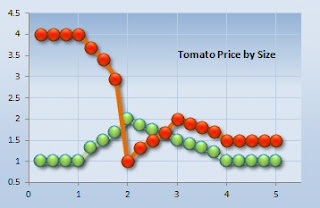So what good is this? Would anyone ever build such a system? Probably not; I’m sure there are better ways to sort tomatoes. But it illustrates one of the general classes of problems for which fuzzy logic is appropriate. So just for fun, let’s imagine how one might us such a system in the real world:

Neil is a tomato buyer for a local food co-op. He buys tomatoes from a large number of small growers, all of whom produce crops of mixed tomatoes, which are harvested sporadically throughout the growing season. The problem is: how to make sure each buyer gets a fair proportion of the return without increasing the overhead too much? Neil notices a bunch of spare parts in his workshop that may help. Using these, he quickly rigs up a small, simple conveyor system – small enough to fit on a pickup truck – for grading the tomatoes. Each tomato rolls over a thin window in the conveyor, where the diminution of light is used to estimate the size of the tomato. It also rolls past two phototransistors, one with a green filter and one with a red, which estimate its color. This information is fed to Neil’s laptop, where the fuzzy logic system defined below is used to estimate the value of the crop. Since the rules are so simple, every couple of weeks, based on discussions with the co-op, the fuzzy logic rules are adjusted to account for the current price of tomatoes. So there it is: all that tomato wisdom, distilled by fuzzy logic into a ketchup of happy farmers, grocers, and customers!

And below is the code. (As always, all the code here is presented "as-is" and without warranty or implied fitness of any kind; use at your own risk.)

`module Fuzzy`
` `
`// The input functions are trapezoids.`
`// The precomputations and closures`
`// make them look more complicated than`
`// they really are.`
` `
`// Technically, since Min and Max are`
`// trapezoids with one side at infinity,`
`// a single function would suffice.`
`// But three functions are more efficient`
`// and comprehensible.`
` `
`// Infinite to the left.`
`let inMin x0 x1 =`
`  let m = 1.0/(x0-x1)`
`  let b = 1.0-x0*m `
`  (fun x ->  `
`     match x<=x0 with`
`     | true -> 1.0`
`     | _ -> `
`     match x<=x1 with`
`     | true -> x*m+b`
`     | _ -> 0.0 )`
` `
`// In the middle.`
`let inMid x0 x1 x2 x3 =`
`  let ml = 1.0/(x1-x0)`
`  let mh = 1.0/(x2-x3)`
`  let bl = 1.0-x1*ml`
`  let bh = 1.0-x2*mh`
`  (fun x -> `
`     match x<x0 with`
`     | true -> 0.0`
`     | _ ->`
`     match x<x1 with`
`     | true -> x*ml+bl`
`     | _ ->`
`     match x<=x2 with`
`     | true -> 1.0`
`     | _ ->`
`     match x<=x3 with`
`     | true -> x*mh+bh`
`     | _ -> 0.0 )`
` `
`// Infinite to the right.`
`let inMax x0 x1 =`
`  let m = 1.0/(x1-x0)`
`  let b = 1.0-x1*m `
`  (fun x ->  `
`     match x>=x1 with`
`     | true -> 1.0`
`     | _ -> `
`     match x>=x0 with`
`     | true -> x*m+b`
`     | _ -> 0.0 )`
` `
`// The output set is a symmetric triangle.`
`// This function returns the area and centroid.`
`let outSym xc dx h = `
`  dx*(2.0-h)*h,xc   `
` `
`// Fire one rule.`
`let fire x (inSet,outSet) =`
`  x |> inSet |> outSet`
` `
`// Fire a rule vector.`
`let fireV xl (inSets,outSet) =`
`  Seq.map2 (fun f x->f x) inSets xl `
`  |> Seq.min`
`  |> outSet `
` `
`// Fire and defuzzify a ruleset.`
`let private fireAll0 f sets x =`
`  List.map (f x) sets `
`  |> List.fold (fun (aa,cc)(a,c)->(aa+a,cc+a*c)) (0.0,0.0)`
`  |> (fun (aa,cc)->cc/aa)`
` `
`// Scalar fire and defuzzify a ruleset.`
`let fireAll sets x = `
`  fireAll0 fire sets x`
` `
`// Vector fire and defuzzify a ruleset.`
`let fireAllV sets x = `
`  fireAll0 fireV sets x`
` `
`// Here's the now familiar tomato problem.`
`// By happy coincidence, all the input sets `
`// can be coded as trapezoids and the output`
`// sets as symmetric triangles.`
` `
`let tiny   = inMin 1.0 2.0 `
`let small  = inMid 1.0 2.0 2.0 3.0`
`let medium = inMid 2.0 3.0 3.0 4.0`
`let large  = inMax 3.0 5.0`
` `
`let green  = inMin 0.0 1.0 `
`let red    = inMax 0.0 1.0 `
` `
`let isGreen = 0.0`
`let isRed   = 1.0`
` `
`let cheap      = outSym 1.00 0.50`
`let moderate   = outSym 1.50 0.50`
`let expensive  = outSym 2.00 0.50`
`let outrageous = outSym 4.00 2.00`
` `
`let rules =`
`  [`
`    ([tiny;green],cheap);`
`    ([small;green],expensive);`
`    ([medium;green],moderate);`
`    ([large;green],cheap);`
` `
`    ([tiny;red],outrageous);`
`    ([small;red],cheap);`
`    ([medium;red],expensive);`
`    ([large;red],moderate);`
`  ]`
` `
`// Inspection will show that the results`
`// are identical to the "tzoid" version.`
` `
`for size in 0.25..0.25..5.00 do`
`  printfn "%f, %f, %f" `
`    size `
`    (fireAllV rules [size;isGreen])`
`    (fireAllV rules [size;isRed])`
` `
`printf "Your breakpoint here."`
` `

## Friday, August 27, 2010

### Fuzzy Logic in F#, Now More Functional!

Before moving forward with some fuzzy logic examples, I decided to take a step back in complexity. I decided that my “tzoid” approach was overkill for 80% of the cool stuff you can do with fuzzy logic. So I came up with something simpler and more functional. But first, a bit more background on fuzzy inference and what I’m trying to achieve.

In its most basic form, fuzzy inference uses at least one pair of fuzzy sets to map a crisp input value onto a new crisp output value. There are a number of ways to do this, but the most common is to take the height of the input set at the input value as x, use that height to truncate the output set, and then “defuzzify” that output set to a crisp value. (Different defuzzification methods work better depending on the domain, but using the centroid is one common method.)

In pseudo-F#, and graphically, this process might look like:

inputValue |> (heightAt inputSet) |> (truncate outputSet) |> defuzzifyThe former is for a single rule. However, most applications require a rule set of a number of rules. The process can be extended by mapping and folding, and then applying some defuzzification function such as a weighted average. Again in pseudo-F#, and graphically, this extended process might look like:

inputValue
|> map ( (heightAt inputSet) >> (truncate outputSet)) ruleset
|> fold defuzzify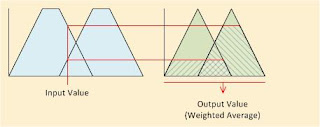And lastly, the input value and input set might be extended to vectors of paired values, each handling a different type of input (e.g. speed, height, acceleration, etc.). These can be combined by some simple method, for example, taking the minimum height for conjunctive rules, the maximum height for disjunctive rules, etc.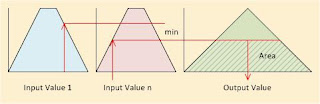So what is the minimal useful implementation of this? Here are some ideas:

1) To start with, I’ll assume a single input set rather than a vector. This will simplify things, and the resulting code will be easy to extend to vectors.

2) Any input set can be described as a simple trapezoid. (Perhaps with an infinite extension on one axis or another.)

3) Any output set can be described as a symmetric triangle. (i.e. an isosceles triangle with its base on the x-axis.)

4) All the sets have a maximum membership value of one.

5) The defuzzification function is a weighted average of truncated output triangle area and centroid.

The code below demonstrates an implementation of this using the tomato price example from an earlier post. Since all the input sets in the original problem were describable as trapezoids and all the output sets were describable as symmetric triangles, the result is identical. Except, of course, there is a lot less source code this time around. (As always, all the code here is presented "as-is" and without warranty or implied fitness of any kind; use at your own risk.)

So what is the “take away”?

It’s not that fuzzy logic is some magic method which yields results not achievable by any other method. The truth is, most problems solvable by fuzzy logic are solvable by other means, such as regression, differential equations, numeric modeling, or other types of rule-based systems. And sometimes, if they are made complex enough or are based on enough data, these other methods can yield more exact results.

What the take away is, is this: fuzzy logic is a conceptually compact and computationally efficient means for transferring imprecise, high-level solutions into precise computer logic.

Very simple, in fact so simple that:

1) Fuzzy logic can be made cheap enough for consumer appliances like microwave ovens, washing machines, and vacuum cleaners.

2) Fuzzy logic can be made reasonably cheap and fast. Fast enough to hundreds or even thousands of inferences per second for things like video cameras and machine control.

3) Fuzzy logic can be made reasonably cheap and parallel. For example, image enhancement for a digital camera by processing entire blocks of pixels. And fuzzy logic is simple enough that it can be implemented on other-purpose parallel hardware – such as graphics adapters – without too much effort. It’s not difficult to imagine an F# implementation of some fuzzy logic system which uses a domain specific language (DSL) and/or F# quotations to interface using CUDA or even High Level Shader Language (HLSL).

`module Fuzzy`
` `
`// Type abbreviations.`
`type InFunc = float->float`
`type OutFunc = float->(float*float)`
` `
`// The input functions are trapezoids.`
`// The precomputations and closures`
`// make them look more complicated than`
`// they really are.`
` `
`// Technically, since Min and Max are`
`// trapezoids with one side at infinity,`
`// a single function would suffice.`
`// But three functions are more efficient`
`// and comprehensible.`
` `
`// Infinite to the left.`
`let inMin x0 x1 =`
`  let m = 1.0/(x0-x1)`
`  let b = 1.0-x0*m `
`  (fun x ->  `
`     match x<=x0 with`
`     | true -> 1.0`
`     | _ -> `
`     match x<=x1 with`
`     | true -> x*m+b`
`     | _ -> 0.0 )`
` `
`// In the middle.`
`let inMid x0 x1 x2 x3 =`
`  let ml = 1.0/(x1-x0)`
`  let mh = 1.0/(x2-x3)`
`  let bl = 1.0-x1*ml`
`  let bh = 1.0-x2*mh`
`  (fun x -> `
`     match x<x0 with`
`     | true -> 0.0`
`     | _ ->`
`     match x<x1 with`
`     | true -> x*ml+bl`
`     | _ ->`
`     match x<=x2 with`
`     | true -> 1.0`
`     | _ ->`
`     match x<=x3 with`
`     | true -> x*mh+bh`
`     | _ -> 0.0 )`
` `
`// Infinite to the right.`
`let inMax x0 x1 =`
`  let m = 1.0/(x1-x0)`
`  let b = 1.0-x1*m `
`  (fun x ->  `
`     match x>=x1 with`
`     | true -> 1.0`
`     | _ -> `
`     match x>=x0 with`
`     | true -> x*m+b`
`     | _ -> 0.0 )`
` `
`// The output function is a symmetric triangle.`
`// This function produces the area and centroid.`
`let outSym xc dx h = `
`  dx*(2.0-h)*h,xc   `
` `
`// Fire one rule.`
`let fire x (inSet:InFunc,outSet:OutFunc) =`
`  x |> inSet |> outSet`
` `
`// Fire and defuzzify a ruleset.`
`let fireAll sets x =`
`  List.map (fire x) sets `
`  |> List.fold (fun (aa,cc)(a,c)->(aa+a,cc+a*c)) (0.0,0.0)`
`  |> (fun (aa,cc)->cc/aa)`
` `
` `
`// Here's the now familiar tomato problem.`
`// By happy coincidence, all the input sets `
`// can be coded as trapezoids and the output`
`// sets as symmetric triangles.`
` `
`let tiny   = inMin 1.0 2.0 `
`let small  = inMid 1.0 2.0 2.0 3.0`
`let medium = inMid 2.0 3.0 3.0 4.0`
`let large  = inMax 3.0 5.0`
` `
`let cheap      = outSym 1.00 0.50`
`let moderate   = outSym 1.50 0.50`
`let expensive  = outSym 2.00 0.50`
`let outrageous = outSym 4.00 2.00`
` `
`let rules =`
`  [`
`    (tiny,outrageous);`
`    (small,cheap);`
`    (medium,expensive);`
`    (large,moderate);`
`  ]`
` `
`// Inspection will show that the results`
`// are identical to the "tzoid" version.`
` `
`for size in 0.25..0.25..5.00 do`
`  printfn "%f, %f" size (fireAll rules size)`
` `
`printf "Your breakpoint here."`

## Thursday, August 26, 2010

### Fuzzy Logic in F#, Example 1

Here is an example of the use of the F# fuzzy logic functions presented yesterday. This example involves the price of tomatoes.

For the purposes of this example, tomatoes come in three sizes: tiny (salad size), small, medium, and large. Of the three larger sizes, people tend to prefer medium, so they are the most expensive. Large (being overripe and watery) are cheaper, and small are the cheapest. However, tiny tomatoes, useful for salads and garnishes, command a premium price.

My solution encodes this tomato wisdom in a set of four rules. It also prints a list of tomato size vs. price, estimated using the rules. I have taken the liberty of graphing this output, complete with gratuitous tomato colorization in the chart below: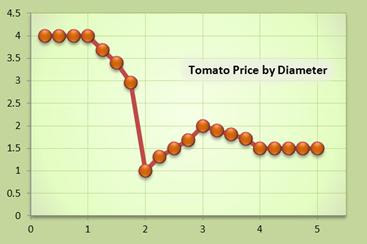And below is the code. It relies on the F# fuzzy logic functions presented in yesterday’s blog. As always, all the code here is presented "as-is" and without warranty or implied fitness of any kind; use at your own risk.

Admittedly, this is not a stunning example of the power of fuzzy logic, but it does illustrate a few of the basic techniques. A good reference, like the previously mentioned Fuzzy Logic With Engineering Applications, by Timothy J. Ross, will reveal the broader range of fuzzy logic techniques.

Tomorrow I will show how conjunction can be used to create rules which use more than one input set to combine different types of data.

`module Program`
` `
`open Trapezoid`
` `
`// This function will turn a pair of`
`// fuzzy sets into a function that will`
`// act as a rule.  The input to the function`
`// is the x value, and the output is a tuple `
`// of the output set area and its centroid.`
`let makeRule (l0,l1) = `
`  let f =`
`    Trapezoid.ofSeq `
`    >> Seq.toArray `
`  let a0 = f l0`
`  let a1 = f l1`
`  (fun x->`
`    Trapezoid.height x a0 `
`    |> Trapezoid.project a1)`
` `
`// Tomato size in inches.`
`let tiny   = [(0.0,1.0);(1.0,1.0);(2.0,0.0)]`
`let small  = [(1.0,0.0);(2.0,1.0);(3.0,0.0)]`
`let medium = [(2.0,0.0);(3.0,1.0);(4.0,0.0)]`
`let large  = [(3.0,0.0);(5.0,1.0);(9.0,1.0)]`
` `
`// Tomato price in \$.`
`let cheap      = [(0.50,0.0);(1.00,1.0);(1.50,0.0)]`
`let moderate   = [(1.00,0.0);(1.50,1.0);(2.00,0.0)]`
`let expensive  = [(1.50,0.0);(2.00,1.0);(2.50,0.0)]`
`let outrageous = [(2.00,0.0);(4.00,1.0);(6.00,0.0)]`
` `
`// The ruleset.`
`let rules =`
`  [`
`    (tiny,outrageous);`
`    (small,cheap);`
`    (medium,expensive);`
`    (large,moderate);`
`  ] |> List.map makeRule`
` `
`// Map the ruleset to a range of`
`// tomato sizes.`
`for size in 0.25..0.25..5.00 do`
`  printfn "%f, %f" `
`    size`
`    (List.map (fun r->r size) rules`
`     |> List.fold (fun (a,b)(ax,bx)->a+ax,b+ax*bx) (0.0,0.0)`
`     |> (fun (a,b)->b/a))`
` `
`printf "Your breakpoint here."`
` `

## Wednesday, August 25, 2010

### Fuzzy Logic Experiments in F#

In an earlier post, I mentioned I was reading Fuzzy Logic With Engineering Applications by Timothy J. Ross. It’s an excellent book, and I’ve been doing some experiments in F# based on my readings in the book. This post outlines some classes and functions I’ve created, and the next post will show a simple test application. (As always, all the code here is presented "as-is" and without warranty or implied fitness of any kind; use at your own risk.)

My basic approach was to code each fuzzy set as a series of right trapezoids. This is not the only approach, and other methods – such as continuous functions or symmetric triangles – may be more appropriate for some situations. I call my trapezoid implementation a “tzoid,” and it’s basic features are shown in the diagram below (refer also to the code at the bottom of this post).A fuzzy set is composed of an ordered collection of tzoids. The collection can be an array, list, sequence, etc., as appropriate; all are considered equivalent for the purposes of defining a set.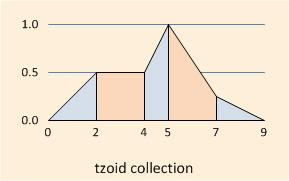The basic fuzzy inference process is this: for each rule, determine the degree of membership of one or more “crisp” values in an antecedent set or sets (“fuzzification”), and use this to estimate the membership in a consequent set. Combine these consequent set memberships and “defuzzify” them into a “crisp” output value.

There are a large number of methods for doing this, each with various pros and cons; I comment you to Fuzzy Logic With Engineering Applications for a detailed discussion. My method here is as follows:

1) Compute the degree of membership of each crisp input parameter as the height of the fuzzy set at that parameter. (Fuzzification.)

2) Combine these degrees of membership using a “min” function. (Conjunction.)

3) Compute the crisp output value by a) truncating each output set at the corresponding height from steps 1 and 2, b) finding the centroid, and c) computing the weighted average (by set area) of all the centroids. (Defuzzification.)

The diagrams below show the operations of finding the height, finding an individual tzoid fractional area (ntroid), and finding a fractional area for an entire fuzzy set (ntroidz). The code shows how the centroidz is found as the ntroidz of half the set area, and a how a “project” function is used to truncate an output set and project a crisp value from its centroid. (There is also a normalize function, which is reserved for future use.)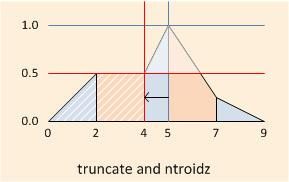That’s it. My next post will show how these functions can be combined to make a simple inference system.

`module Trapezoid`
` `
`open System.Collections.Generic`
` `
`// Defines a right trapeziodal area.`
`// (Trapezoid is called trapezium in British English, IIRC.)`
`[<System.Diagnostics.DebuggerDisplayAttribute("{X0},{Y0}-{X1},{Y1}")>]`
`type tzoid =`
`  {`
`    X0 : float;`
`    Y0 : float;`
`    X1 : float;`
`    Y1 : float;`
`    Slope : float;`
`    Intercept : float;`
`  }`
`  member this.X y =`
`    match this.Slope with `
`    | 0.0 -> this.X0 `
`    | _ -> (y-this.Intercept)/this.Slope`
`  member this.Y x =`
`    x*this.Slope+this.Intercept`
`  member this.Area =`
`    (this.X1-this.X0)*(this.Y0+this.Y1)/2.0`
`  static member Make x0 y0 x1 y1 = `
`    let slope = (y1-y0)/(x1-x0) `
`    {`
`      X0=x0; Y0=y0; X1=x1; Y1=y1;`
`      Slope=slope;`
`      Intercept=y0-x0*slope;`
`    }`
` `
`// Turns a sequence of pairs into`
`// a sequence of tzoids. `
`let ofSeq s =`
`  s`
`  |> Seq.pairwise `
`  |> Seq.map (fun ((x0,y0),(x1,y1))->tzoid.Make x0 y0 x1 y1)`
` `
`// Returns true if the tops of the tzoids are colinear.`
`let colinear (t0:tzoid) (t1:tzoid) =`
`  (t0.Slope=t1.Slope)&&(t0.Intercept=t1.Intercept)`
` `
`// Truncates a tzoid, possibly splitting it.`
`// Designed for use with fold functions.`
`let truncate y tr acc =`
`  match ((y<tr.Y0)&&(y>tr.Y1))||((y>tr.Y0)&&(y<tr.Y1)) with`
`  | true -> `
`    let xn = tr.X y`
`    (tzoid.Make tr.X0 (min tr.Y0 y) xn y)::`
`    (tzoid.Make xn y tr.X1 (min tr.Y1 y))::`
`    acc`
`  | false -> `
`    (tzoid.Make tr.X0 (min y tr.Y0) `
`                tr.X1 (min y tr.Y1))::acc`
` `
`// Normalize a tzoid by combining colinear neighbors.`
`// Designed for use with fold functions.`
`let normalize tr acc =`
`  match acc with `
`  | h::t ->`
`    match colinear h tr with`
`    | false -> tr::acc`
`    | true -> (tzoid.Make tr.X0 tr.Y0 h.X1 h.Y1)::t`
`  | [] -> [tr]`
` `
`// X value for a given area (a) in a tzoid.`
`let ntroid x0 x1 y m a = `
`  match m with`
`  | 0.0 -> `
`    match y with`
`    | 0.0 -> (x0+x1)/2.0`
`    | _ -> a/y+x0 `
`  | _ -> x0+(sqrt(y*y+2.0*a*m)-y)/m`
` `
`// X value for a given area (a) in`
`// a list of tzoids.`
`let ntroidz a (l:tzoid list) =`
`  let rec f acc (l:tzoid list) =`
`    match l with `
`    | h::t -> `
`      let acc0 = h.Area+acc`
`      match acc0>=a with`
`      | true -> ntroid h.X0 h.X1 h.Y0 h.Slope (a-acc)`
`      | _ -> f acc0 t `
`    | _ -> System.Double.NaN`
`  match a with`
`  | 0.0 ->`
`    match l with `
`    | [] -> System.Double.NaN `
`    | h::t -> `
`      (h.X0+(List.nth l ((List.length l)-1)).X1)/2.0`
`  | _ -> f 0.0 l`
` `
`// Centroid X value for a list of tzoids.`
`let centroidz (l:tzoid list) =`
`  let a = List.sumBy (fun (t:tzoid)->t.Area) l`
`  a,(ntroidz (a/2.0) l)`
` `
`// Garden-variety binary search.`
`let inline bsearch (cmp:'V0->int) (a:'V0[]) =`
`  let rec f lo hi =`
`    match lo>hi with`
`    | true -> None`
`    | _ ->`
`      let mid = lo+(hi-lo)/2`
`      match cmp a.[mid] with`
`      | 0 -> Some(a.[mid])`
`      | x when x<0 -> f (mid+1) hi`
`      | _ -> f lo (mid-1)`
`  f 0 (a.Length-1)`
` `
`// Find height at x in a list of tzoids.`
`let height x (a:tzoid[]) =`
`  let f (t:tzoid) = `
`    match x<t.X0 with`
`    | true -> 1`
`    | _ ->`
`    match x>t.X1 with`
`    | true -> -1`
`    | _ -> 0`
`  match bsearch f a with`
`  | None -> 0.0`
`  | Some(t) -> t.Y x`
` `
`// Truncate a tozid array at height h`
`// and return the new centroid.`
`let project (a:tzoid[]) h =`
`  Array.foldBack (truncate h) a []`
`  |> centroidz`